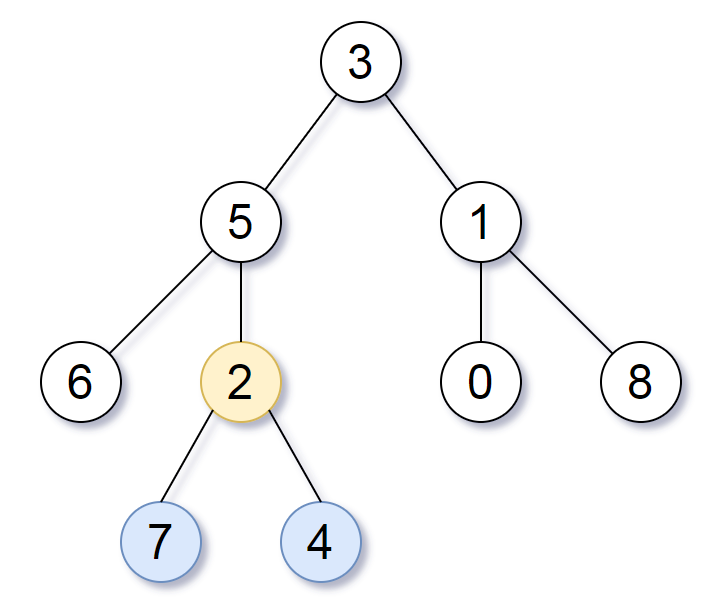865. Smallest Subtree with all the Deepest Nodes

Given the `root` of a binary tree, the depth of each node is the shortest distance to the root.

Return the smallest subtree such that it contains all the deepest nodes in the original tree.

A node is called the deepest if it has the largest depth possible among any node in the entire tree.

The subtree of a node is tree consisting of that node, plus the set of all descendants of that node.

Note: This question is the same as 1123: https://leetcode.com/problems/lowest-common-ancestor-of-deepest-leaves/

Example 1:```Input: root = [3,5,1,6,2,0,8,null,null,7,4]
Output: [2,7,4]
Explanation: We return the node with value 2, colored in yellow in the diagram.
The nodes coloured in blue are the deepest nodes of the tree.
Notice that nodes 5, 3 and 2 contain the deepest nodes in the tree but node 2 is the smallest subtree among them, so we return it.
```

Example 2:

```Input: root = 
Output: 
Explanation: The root is the deepest node in the tree.
```

Example 3:

```Input: root = [0,1,3,null,2]
Output: 
Explanation: The deepest node in the tree is 2, the valid subtrees are the subtrees of nodes 2, 1 and 0 but the subtree of node 2 is the smallest.
```

Constraints:

• The number of nodes in the tree will be in the range `[1, 500]`.
• `0 <= Node.val <= 500`
• The values of the nodes in the tree are unique.

865. Smallest Subtree with all the Deepest Nodes
``````struct Solution;
use rustgym_util::*;

trait Postorder {
}

fn postorder(&self) -> (usize, TreeLink) {
use std::cmp::Ordering::*;
if let Some(node) = self {
let node = node.borrow();
let left = &node.left;
let right = &node.right;
let (left_depth, left_tree) = left.postorder();
let (right_depth, rigth_tree) = right.postorder();
match left_depth.cmp(&right_depth) {
Equal => (left_depth + 1, self.clone()),
Less => (right_depth + 1, rigth_tree),
Greater => (left_depth + 1, left_tree),
}
} else {
(0, None)
}
}
}

impl Solution {
root.postorder().1
}
}

#[test]
fn test() {
let root = tree!(
3,
tree!(5, tree!(6), tree!(2, tree!(7), tree!(4))),
tree!(1, tree!(0), tree!(8))
);
let res = tree!(2, tree!(7), tree!(4));
assert_eq!(Solution::subtree_with_all_deepest(root), res);
}
``````